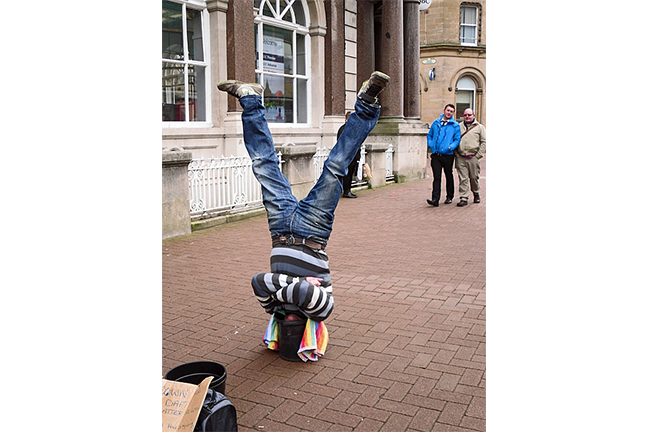## Want to keep learning?

This content is taken from the UNSW Sydney's online course, Maths for Humans: Linear, Quadratic & Inverse Relations. Join the course to learn more.
3.9

## UNSW SydneyAn inverse view

# Inverse proportionalities

We’ve looked at linear relations, and quadratic relations. Now it is time to look at a quite different kind of connection: an inverse relation between variable quantities $$\normalsize{x}$$ and $$\normalsize{y}$$ given by the equation $${y=\dfrac{a}{x}}$$ for some fixed number $$\normalsize a\gt 0$$. This connects directly with the geometry of the hyperbola that we have been studying.

In this step, we introduce inverse relations and discuss basic examples.

## Inverse relations and symmetry

An inverse relation, or inverse proportionality is a relation of the form

$\Large{y=\frac{a}{x}}$

for some fixed constant of proportionality $$\normalsize a\gt 0$$. In this kind of situation, the bigger $$\normalsize{x}$$ gets the smaller $$\normalsize{y}$$ gets, and conversely, the smaller $$\normalsize{x}$$ gets the larger $$\normalsize{y}$$ gets. In fact by thinking about the particular inverse relation $$\normalsize{y=1/x}$$ we see that the two intervals $$\normalsize{(0,1]}$$ and $$\normalsize{[1,\infty)}$$ can be completely matched up: for every number in the first interval, its reciprocal is a number in the second interval, and vice versa. Of course this matching severely distorts measurements.

If we rewrite the basic inverse relation in the form

$\Large{xy=a}$

we see that it is completely symmetric between $$\normalsize{x}$$ and $$\normalsize{y}$$: the product of $$\normalsize{x}$$ and $$\normalsize{y}$$ is constant.

Also we see that if one of $$\normalsize{x}$$ and $$\normalsize{y}$$ is positive, so is the other one. Conversely if one is negative, then so is the other one. We can also state the relation in the form

$\Large y \propto \frac{1}{x}.$

## Speed and time

The familiar equation

$\Large{\operatorname{speed}=\frac{\operatorname{distance}}{\operatorname{time}}}$

expresses the fact that if $$\normalsize{\operatorname{distance}}$$ is constant, then $$\normalsize{\operatorname{speed}}$$ and $$\normalsize{\operatorname{time}}$$ are inversely proportional. In this discussion the terms velocity and speed are essentially synonymous.

For example suppose we want to travel $$\normalsize{10}\;\text{km}$$. If we have a speed of $$\normalsize{50\;\text{km}/\text{hr}}$$, then the time taken will be $$\normalsize{10/50=1/5}$$ hours, or $$\normalsize{12}$$ minutes. If we have a speed of $$\normalsize{100\;\text{km}/\text{hr}}$$, then the time taken will be only $$\normalsize{6}$$ minutes. If we double one quantity, the other halves. If we triple one quantity, the other is divided by three, and so on.

Q1 (E): If an Olympic walker takes $$\normalsize{3}$$ hours to walk a race, and I walk half as fast as the Olympic walker, then how long will it take me to walk the same race?

Q2 (E): If a marathon runner runs $$\normalsize{42}$$ kilometers in three hours, and you run $$\normalsize{5}$$ kilometers in $$\normalsize{45}$$ minutes, then what is the ratio between your average speed and the marathon runner’s average speed?

## Many hands make light work

Suppose we have a major construction project, involving moving lots of bricks from one place to another.Concrete blocks By Justanother GFDL or CC BY-SA 3.0, via Wikimedia Commons

Suppose it takes $$\normalsize{5}$$ people $$\normalsize{8}$$ hours to move the full load of bricks. We’ll suppose that moving bricks is an individual process, not requiring any significant cooperation. It follows that $$\normalsize{10}$$ people ought to complete the task in half the time, namely $$\normalsize{4}$$ hours. If we had $$\normalsize{20}$$ people, we could half the time again, to $$\normalsize{2}$$ hours. On the other hand, a single person would probably take about $$\normalsize{5 \times 8=40}$$ hours.

This is a good example of an inverse relation, between the number of people $$\operatorname{N}$$ to do the job, and the time $$\operatorname{T}$$ taken to do the job. And what is the specific relationship here? In this case the relationship between $$\operatorname{N}$$ and $$\operatorname{T}$$ is the inverse relation

$\Large \operatorname{T}=\frac{40}{\operatorname{N}}.$

Another way to state this is $$\operatorname{T}\times \operatorname{N}=40$$, which can be interpreted as saying that the job takes a total of 40 person-hours.

Q3 (M): If $$\normalsize{8}$$ people take $$\normalsize{5}$$ hours to paint a house, how long would it take $$\normalsize{3}$$ people to paint the same house?

The next question is a bit trickier!egg, By Hans, Free for commercial use / No attribution required

Q4 (C): If $$\normalsize{6}$$ children take $$\normalsize{5}$$ hours to paint $$\normalsize{4}$$ Easter eggs, then how long would it take $$\normalsize{5}$$ children to paint $$\normalsize{3}$$ Easter eggs?

A1. Since we halve the speed, the time doubles, and so it will take $$\normalsize{6}$$ hours to walk the same race.
A2. The average speed of the marathon runner is $$\normalsize{\frac{42\;\text{km}}{3\;\text{hr}}=14\;\text{km}/\text{hr}}$$. My average speed is $$\normalsize{\frac{5\;\text{km}}{45\;\text{min}}}$$, or equivalently, $$\normalsize{\frac{5\;\text{km}}{3/4\;\text{hr}}=20/3 \;\text{km}/\text{hr}}.$$ Therefore, the ratio of average speeds is $$\normalsize{\frac{20/3}{14}}=10/21.$$
A3. A single person will paint the house in $$\normalsize{8\times 5\;\text{hr}=40\;\text{hr}}.$$ Therefore, three people will paint the same house in $$\normalsize{40/3}\approx 13.3$$ hours.
A4. One child will paint $$\normalsize{4}$$ eggs in $$\normalsize{6\times 5\;\text{hr}=30\;\text{hr}}$$. Therefore, one child will paint one egg in $$\normalsize{30/4\;\text{hr}=15/2\;\text{hr}}$$. Thus, $$\normalsize{5}$$ children will paint one egg in $$\normalsize{\frac{15/2}{5}\;\text{hr}=3/2\;\text{hr}}$$ (this is an inverse relation), and so $$\normalsize{5}$$ children will paint $$\normalsize{3}$$ eggs in $$\normalsize{3\times 3/2\;\text{hr}=9/2\;\text{hr}}$$ (this is a direct relation).